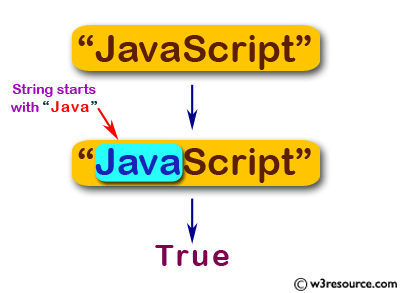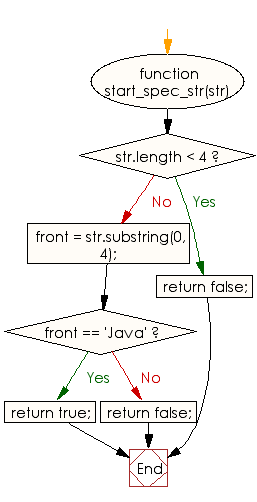# JavaScript: Check whether a string starts with 'Java' and false otherwise

## JavaScript Basic: Exercise-27 with Solution

Write a JavaScript program to check whether a string starts with 'Java' and false otherwise.

Pictorial Presentation:Sample Solution:

HTML Code:

``````<!DOCTYPE html>
<html>
<meta charset="utf-8">
<meta name="viewport" content="width=device-width">
<title>JavaScript program to check whether a string starts with 'Java' and false otherwise.</title>
<body>
</body>
</html>
```
```

JavaScript Code:

``````function start_spec_str(str)
{
if (str.length < 4)
{
return false;
}
front = str.substring(0, 4);
if (front == 'Java')
{
return true;
}
else
{
return false;
}
}

console.log(start_spec_str("JavaScript"));
console.log(start_spec_str("Java"));
console.log(start_spec_str("Python"));
```
```

Sample Output:

```true
true
false
```

Flowchart:ES6 Version:

``````function start_spec_str(str)
{
if (str.length < 4)
{
return false;
}
front = str.substring(0, 4);
if (front == 'Java')
{
return true;
}
else
{
return false;
}
}

console.log(start_spec_str("JavaScript"));
console.log(start_spec_str("Java"));
console.log(start_spec_str("Python"));
``````

Live Demo:

See the Pen JavaScript: check if a string starts with Java -basic- ex-27 by w3resource (@w3resource) on CodePen.

Improve this sample solution and post your code through Disqus

What is the difficulty level of this exercise?

Test your Programming skills with w3resource's quiz.

﻿

## JavaScript: Tips of the Day

Returns the sum of the powers of all the numbers from start to end (both inclusive)

Example:

```const sumPower = (end, power = 2, start = 1) =>
Array(end + 1 - start)
.fill(0)
.map((x, i) => (i + start) ** power)
.reduce((a, b) => a + b, 0);
console.log(sumPower(10)); // 385
console.log(sumPower(10, 3)); // 3025
console.log(sumPower(10, 3, 5)); // 2925
```

Output:

```385
3025
2925
```